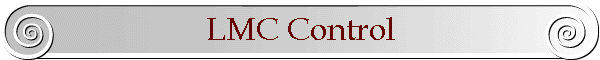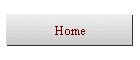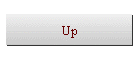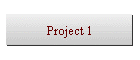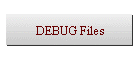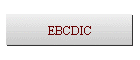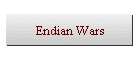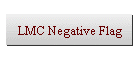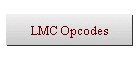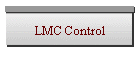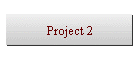Updated: 2003-09-23 11:44

# Coding LMC Control Flow

Most programs contain some form of conditional logic, e.g. IF statements that affect the usual linear flow of control in the program.  Programming conditional logic means turning the pseudocode logic into statements written in mnemonic form for the Little Man Computer (LMC).  The LMC has no IF, WHILE, FOR or other structured statements; we must use the limited control flow instructions available to us to implement structured code.

High-level language conditional statements contain a test expression that returns a TRUE or FALSE, e.g. IF (count == 0), WHILE (sum >= MAX), etc.  The simplest test expression is a test that compares two quantities, e.g. A<B.  Let's name these two quantities with variable names A and B and work out the translation of two-variable test expressions to LMC instructions.  More complex expressions can be built using the techniques developed here.

## IF and WHILE Statements

Note that the test in an IF statement and the test in a WHILE statement have exactly the same structure; both expressions return TRUE or FALSE and alter the flow of control around an enclosed body of statements:

```if ( a == b ) then
a = a + b    // TRUE body
endif```
```while ( a == b ) do
a = a + b    // TRUE body
endwhile```

In both the above code fragments, the control flow enters the TRUE body only if the test expression comparing A and B for equality is true. If we know how to code one test in LMC instructions, we know how to code the other.  We need only focus on the code for the test expression itself.

## Basic IF Structure

The basic LMC instructions that alter the flow of control are SKIP and JUMP statements.  We must use these two LMC instructions to implement our pseudocode IF and WHILE control flow statements.

The pseudocode structure for a simple IF statement (no ELSE clause) is as follows:

```	// Simple IF statement structure pseudocode:
Evaluate the test expression using A and B
SKIP the next statement if the test is true
Perform the many
statements that are
the body of the IF here...
ENDIF:	Continue with rest of program```

Note the placement of the SKIP and JUMP instructions, above.  The function of the JUMP is to get around the body of the IF statement.  The SKIP statement precedes the JUMP and controls whether or not the JUMP is taken.  We must code a test expression and choose a SKIP statement that transfers flow of control into the body of the IF only if the test expression is TRUE.  If the test expression is FALSE, the JUMP should take control around the body of the IF statement.

## Mathematics, Lights, and SKIP

Each type of SKIP instruction checks the settings of one of the three LMC flag lights: Negative, Zero, or Positive.  Only mathematics, i.e. an ADD or SUBTRACT, sets the LMC lights; therefore, to use SKIP statements to alter the flow of control in our program, we must first perform an ADD or SUBTRACT using our two variables A and B.  The mathematics will set the LMC lights based on the relationship between A and B, and we can then SKIP according to the setting of the lights.

For example, consider the following code fragment:

```if ( a == b ) then
a = a + b    // TRUE body
endif
ouput a```

We have an expression above that causes control flow to enter the TRUE body only if A is equal to B.  Using the IF statement structure given above, we need to pick some mathematics to set the flag lights, follow the math with a SKIP instruction, and follow the SKIP instruction with a JUMP instruction.  Using that structure, here is a translation of the above pseudocode into LMC instructions:

```	LDA	A	; do mathematics on A and B...
SUB	B	;   ...to set the lights
SKZ		; SKIP *into* IF body if A == B
JMP	ENDIF	; A is not equal to B - jump around
LDA	A	; body of IF...
ADD	B	;   body of IF...
STO	A	;     body of IF.
ENDIF	LDA	A	; end of IF statement - continue on
OUT```
If A and B are equal, subtracting them will cause the Zero flag to come on.  The SKZ instruction will then operate to skip over the JUMP instruction.  In other words, the SKZ causes a skip into the body of the IF statement.  If A and B are not equal, the Zero flag will not come on, and the SKZ will do nothing.  The JMP will jump around the body of the IF statement.

All LMC flow control statements (IF, WHILE) can be programmed using the above structure:

1. Do some mathematics using A and B to set the LMC flag lights.
2. Use one of the SKIP instructions to skip the next JUMP instruction.
3. Use a JUMP instruction to jump around the body of the IF or WHILE.

How do we choose what mathematics to perform, and which SKIP instruction to use?  We will examine that later in this document.

## IF with an ELSE clause

IF statements with ELSE clauses are only slightly more complex than simple IF statements.  Here is a pseudocode program fragment using an IF/ELSE statement:

```1. if ( a == b ) then
2.     a = a + b
3. else
4.     b = b - a
5. endif
6. stop```

Here is a translation of the above pseudocode fragment into LMC assembler, with the statement numbers inserted as comments:

```	LDA	A	; 1. start IF: test ( a == b )
SUB	B
SKZ
JMP	ELSE
LDA	A	; 2. body of TRUE part of IF
STO	A
JMP	ENDIF
ELSE	LDA	B	; 3,4. body of FALSE part of IF
SUB	A
STO	B
ENDIF	HLT		; 5,6. ENDIF; after IF```
Compare the above LMC code with that used in the simple IF statement.  Note the identical placement of the test expression mathematics, the SKIP, and the JUMP instruction on line 1, above.  The function of the first JUMP is still to get around the TRUE body of the IF statement; however, instead of jumping to the end of the IF statement, it now jumps to the first statement in the ELSE clause.  (If the test expression of an IF/ELSE statement is FALSE, control passes over the TRUE body and into the FALSE body.)

A new "JMP ENDIF" instruction has been added to the end of the TRUE body of the IF statement (just before the ELSE label) to jump around the FALSE body that makes up the ELSE part of the IF.  If this jump were not there, the flow of control would run from the statements at the end of the TRUE body into the statements at the beginning of the FALSE body.

## A WHILE Statement

The following pseudocode fragment is almost identical to the simple IF example used earlier; only the keywords IF have been changed to WHILE:

```while ( a == b ) then
a = a + b    // TRUE body
endwhile
ouput a```

Again, we have written an expression that causes control flow to enter the TRUE body only if A is equal to B.  We need to pick some mathematics to set the flag lights, follow the math with a SKIP instruction, and follow the SKIP instruction with a JUMP instruction.  We use almost the same statement structure as for the simple IF statement.  Here is a translation of the above pseudocode into LMC instructions:

```TEST	LDA	A	; do mathematics on A and B...
SUB	B	;   ...to set the lights
SKZ		; SKIP *into* WHILE body if A == B
JMP	ENDWH	; A is not equal to B - jump around
LDA	A	; body of WHILE...
ADD	B	;   body of WHILE...
STO	A	;     body of WHILE.
JMP	TEST	; WHILE is a loop - jump to top to start over
ENDWH	LDA	A	; end of WHILE statement - continue on
OUT```
The analysis of the above LMC code is similar to the analysis of the code for the simple IF statement:  If A and B are equal, subtracting them will cause the Zero flag to come on.  The SKZ instruction will then operate to skip over the JUMP instruction.  In other words, the SKZ causes a skip into the body of the WHILE statement.  If A and B are not equal, the Zero flag will not come on, and the SKZ will do nothing.  The JMP will jump around the body of the WHILE statement.

The key difference between the above code and the code for the simple IF statement is the addition of a "JMP TEST" instruction at the bottom of the body of the WHILE statement.  WHILE statements need to loop; IF statements do not.  The jump returns control to the top of the WHILE loop, which is to the test expression that begins the loop.

## FOR Statements

FOR statements are simply a shorthand way of writing the equivalent WHILE statements.  To code a FOR statement, turn it into the equivalent WHILE statement and then translate that into assembler:

```	// the original FOR statement
for ( i=0; i < 10; i++ ) do
x = x + i
endfor```
```	// translated into a WHILE statement
i = 0
while ( i < 10 ) do
x = x + i
i = i + 1
endwhile```
```	; turned into LMC assembler
LDA	ZERO	; ZERO is a mailbox containing constant 000
STO	I
TEST	LDA	I	; do mathematics to set the lights
SUB	TEN	; TEN is a mailbox containing constant 010
SKN		; SKIP into WHILE body if I < 10
JMP	ENDWH	; I is >= 10 - jump around
LDA	X	; body of WHILE...
ADD	I	;   body of WHILE...
STO	X	;     body of WHILE.
LDA	I	; increment...
STO	I	;     increment.
JMP	TEST	; WHILE is a loop - start over
ENDWH	...		; end of WHILE statement - continue on```

The Initialization clause of the FOR loop precedes the WHILE statement.  The body of the WHILE loop ends with the Increment clause of the FOR loop, just before jumping back to the top of the loop.  The WHILE loop itself has exactly the same structure as before:

1. Do some mathematics to set the LMC flag lights.
2. Use one of the SKIP instructions to skip the next JUMP instruction.
3. Use a JUMP instruction to jump around the body.

A common error when programming FOR loops is to mis-code the jump to the top of the loop.  Some people mistakenly code the jump to go to the first statement of the Initialization clause.  The correct jump is to the first statement of the Test part of the WHILE loop, not to the Initialization clause.

## Choosing the Mathematics and SKIP

All of the above control structures (IF, IF/ELSE, WHILE, FOR) have the same structure for their test conditions:

1. Do some mathematics on A and B to set the LMC flag lights.
2. Use one of the SKIP instructions to skip the next JUMP instruction.
3. Use a JUMP instruction to jump around a body.

How do we choose what mathematics to perform using A and B, and which SKIP instruction do we use in front of the JUMP instruction?

Since we are testing the relationship between A and B - equal, less than, greater than, etc. - the operation that works best to set the LMC flag lights is SUBTRACT.  Subtracting A from B or B from A sets the lights according to the relative values of A and B; ADDING would not have the same effect.  The only issues to resolve are whether to do A-B or B-A to set the lights, and which of the few SKIP instructions we need to use.

### LMC Flag Lights Table

Here is a table of LMC flag lights that will help us choose the order of the subtraction and which SKIP to use.  The intersection of a row and column gives the flag lights that come on when A and B are subtracted from each other and have the relationship to each other given in the column heading:

Table of
LMC Lights
Actual relationship of A and B
A < B A == B A > B
Order
of
Subtraction
A - B N NZ Z P P NZ
B - A P NZ Z P N NZ

For example, if we were to perform subtraction "A - B", the first row of the table applies.  For A-B the table shows that if A were less than than B (the first column in the table) the Negative and NonZero lights would come on.  There is no "NonZero" light; but, we do have an extended "skip if non zero" SKNZ instruction that skips if the Zero light is off.  To express this feature in the table, we invent a fictitious NonZero flag "NZ" that is "on" whenever the Zero light is off.  The NZ flag will be on whenever A and B are not equal; it doesn't matter which way we subtract them.

If we were to perform the subtraction in the reverse order, "B - A", the second row of the table applies.  For B-A the table shows that if A were larger than B (column 3 in the table) the Negative light would come on and the fictitious NonZero flag "NZ" would also be on.

To pick a subtraction order and SKIP instruction for a conditional expression, we compare the condition in the expression with the conditions at the top of the columns in the table.  We seek to find an LMC flag light that is ON only in the column or columns that match our conditional expression.  We code our SKIP instruction to skip into the body of our statement based on that flag light.

### Example 1 using the table

A < B A == B A > B
A - B N NZ Z P P NZ
B - A P NZ Z P N NZ

For example, suppose we want to pick the subtraction order and the SKIP instruction for this test expression:

`if ( a > b ) then ...`

The expression A>B matches column 3 in the table.  To set the lights we need and choose the correct SKIP instruction, we must choose between subtraction order A-B (row 1) and subtraction order B-A (row 2):

1. Row 1: A-B: If we try row 1, subtraction order A-B, we see that the Positive and Non-Zero flags are set when we do A-B and A is greater than B.  We might think of programming our SKIP instruction to be either SKP or SKNZ; either one would produce a skip into the body of our statement if A were actually greater than B.  However, note that in this row of the table the Positive light also comes on even if A is equal to B (column 2), so using SKP would also cause an incorrect execution of our statement body if A were equal to B.  So we can't skip using the Positive light.  Similarly, we can't use the NonZero flag (as in SKNZ), since it would skip into the body of our statement both if A were greater than B (column 3) or if A were less than B (column 1).

There is no LMC flag in this A-B row that is on only for A>B and off for the other two columns.  This rules out using any of the lights set using subtraction in the order A-B in row 1; we must try the other order in row 2 of the table.
2. Row 2: B-A: If we try row 2, subtraction order B-A, we see that the Negative and NonZero flags are set when we do B-A and A is greater than B.  We can't skip using the NonZero flag, since the flag also comes on in this row if A is less than B.  However, the Negative light comes on in this row only if A is greater than B.  The Negative light is on only in the A>B column.  This is exactly what we need - the second row has the correct subtraction order we need (row B-A), the column containing the condition we want is the third column (column A>B), and the skip instruction to use will be SKN to select the Negative light:
```	LDA	B	; subtract B-A (second row of table)
SUB	A
SKN		; choose the Negative light to skip into the body for A>B
JMP	ENDIF```

### Example 2 using the table

A < B A == B A > B
A - B N NZ Z P P NZ
B - A P NZ Z P N NZ

For a second example, suppose we want to pick the subtraction order and the SKIP instruction for this test expression:

`while ( a <= b ) do ...`

The expression A<=B doesn't match any single column in the table.  We need to satisfy two columns for this condition: the A<B column (column 1) or the A==B column (column 2).  We need a flag that is ON in both of these columns, and off in the other column.  We again examine both rows of the table to pick the correct subtraction order and correct lights to check.

By looking at the table, we see that the Positive light in the second row (B-A) is the only flag that satisfies our requirements. The Positive light comes on in the second row only if A is less than B or if A is equal to B.  This is exactly what we need - the second row is the correct subtraction order we need (B-A) and the skip instruction to use will be SKP to select the Positive light:

```	LDA	B	; subtract B-A (second row of table)
SUB	A
SKP		; choose the Positive light to pick A<B or A==B
JMP	ENDWHILE```

### Example 3 using the table

A < B A == B A > B
A - B N NZ Z P P NZ
B - A P NZ Z P N NZ

Here is a third example:

`while ( a != b ) do ...`

The expression A!=B doesn't match any column in the table.  Since another way of saying "is not equal" is to say "is greater than or is less than", we actually need to satisfy two columns for this condition: the A<B column (column 1) or the A>B column (column 3).  We need a flag that is ON in these two columns, and is off everywhere else.

We eventually settle on the fictitious flag NZ (NonZero) as being on in columns 1 and 3 and off in column 2.  The order of subtraction doesn't matter; both rows are identical with respect to the NZ flag.  We pick the first row (A-B), and the code fragment we write is:

```	LDA	A	; subtract A-B (first row of table)
SUB	B
SKNZ		; choose the NonZero pseudo-flag to pick A<B or A>B
JMP	ENDWHILE```

### Nested AND Logic

Here is a more complex example using two test expressions joined by AND:

```if ( a != b && c >= d ) then
... body goes here ...
endif```

The above test expression is not a simple one involving two quantities, so the methods we have been using so far will not work directly.  However, we can rewrite the above compound test expression into two nested IF statements, each of which uses a simple A/B-type expression that we know how to translate:

```if ( a != b ) then
if ( c >= d ) then
... IF body goes here ...
endif
endif
... linear code resumes here ...```
Since the IF statements are nested, the body will not be executed unless both the outer AND inner IF conditions are both true.  We can now translate these two IF statements directly into LMC assembler:
```    	LDA	A	; this test is the outer IF
SUB	B
SKNZ
JMP	ENDIF1```
```    	LDA	C	; this test is the inner IF
SUB	D
SKP
JMP	ENDIF2
... IF body goes here ...
ENDIF2
ENDIF1	... linear code resumes here ...```

Both ENDIF1 and ENDIF2 are actually labels for the same location; jumping to either label jumps to the same code at the end of the IF statement.  We might therefore simplify the code and use only a single ENDIF label for both jumps.

### Nested OR Logic

Here is another complex example, this time using two expressions joined by OR:

```if ( a != b || c >= d ) then
... IF body goes here ...
endif```

The above two conditional test expressions are joined by OR, so we cannot implement a solution using two nested IF statements as we did when they were joined by AND.

With a bit of help from deMorgan's theorem in Boolean logic, we can invert the condition so that it becomes one containing AND instead of OR, and then use the inverted condition to branch around the loop body:  (We use the inverted condition to branch around, so the if the condition fails, we enter the body, as desired.)  We are going to turn this original statement:

```if ( CONDITION ) then
... IF body goes here ...
endif```

into this equivalent code using an inverted ("NOT") condition:

```        if ( NOT CONDITION ) then
goto around
endif
... IF body goes here ...
around: ... linear code resumes here ...```

First, we must invert the condition, then apply deMorgan's theorem.  Doing so will turn the condition into one that uses AND instead of OR:

```original condition:  a != b || c >= d
inverted condition:  !(a != b || c >= d)
deMorgan applied:    !(a != b) && !(c >= d)
simplified:          a == b && c < d```
We then use that new inverted condition to branch around the loop body:
```	if ( a == b && c < d ) then
goto around
endif
... IF body goes here ...
around:	... linear code resumes here ...```
We now have a compound condition joined by AND, a problem we can solve with nested IF statements, as we did in the previous section.  The GOTO becomes a simple JUMP statement in LMC assembler:
```    	LDA	A	; this test is the outer IF
SUB	B	;   that tests a == b
SKZ
JMP	ENDIF```
```    	LDA	C	; this test is the inner IF
SUB	D	;   that tests c < d
SKN
JMP	ENDIF
JMP	AROUND
ENDIF	... IF body goes here ...
AROUND	... linear code resumes here ...```
Unlike previous examples, where the JUMP to ENDIF branched around the IF body, the above code uses an inverted condition and the ENDIF labels lead to the IF body.

You might observe that our solution generated a SKN preceding two JMP instructions, one of which only jumps one instruction.  If we were concerned about memory usage, the code might be optimized (at the expense of clarity) by inverting the SKN  to SKP and removing one of the JUMPs:

```    	...
LDA	C	; this test is the inner IF
SUB	D	;   that now tests c >= d
SKP
JMP	AROUND
ENDIF	... IF body goes here ...
AROUND	... linear code resumes here ...```
Making this optimization makes it harder to see the relationship between the code and the pseudocode.  Unless memory space is tight, do not optimize your code.  Make it right, first!

## Do/While Loops

DO/WHILE loops have this structure, with the conditional test at the bottom of the loop:

```do {
a = a + b;    // TRUE body
} while ( a == b );
ouput a```

Unlike the IF statement and WHILE statement, the TRUE body of a DO/WHILE is always executed at least once.  We have a conditional expression that causes control flow to repeat the TRUE body only if A is equal to B.  As before, we will need to pick some mathematics to set the flag lights, follow the math with a SKIP instruction, and follow the SKIP instruction with a JUMP instruction.  Unlike before, the JUMP instruction does not jump out of the loop, it jumps back into the loop.  This means that everything we've done for IF and WHILE statements to choose the order of subtraction and the SKIP instruction is backwards for DO/WHILE statements.  We have to invert the condition to make the DO/WHILE loop work; because, the JUMP must jump into the loop, not out of the loop.

The condition at the end of the above DO/WHILE loop is "A==B".  To code it in LMC assembler, we must pick a subtraction order and SKIP that implements the inverted condition: "A!=B".  We consult the LMC Flag Light Table to do that.  Here is a translation of the above pseudocode into LMC instructions:

```LOOP	LDA	A	; body of DO/WHILE...
ADD	B	;   body of DO/WHILE...
STO	A	;     body of DO/WHILE.
LDA	A	; do mathematics on A and B...
SUB	B	;   ...to set the lights
SKNZ		; SKIP *out of* DO/WHILE loop if A != B
JMP	LOOP	; DO/WHILE is a loop - jump to top to start over
LDA	A	; end of WHILE statement - continue on
OUT```
If A and B are equal, subtracting them will cause the Zero flag to come on.  The SKNZ instruction will do nothing, and the JUMP instruction will repeat the loop.  When A and B are not equal, the SKNZ instruction will detect this, skip over the JUMP, and cause the loop to exit.  In other words, the SKNZ causes a skip out of the body of the DO/WHILE loop.  (The SKIP caused a skip into the body of a WHILE loop.)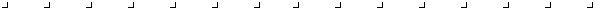Web Author: Ian! D. Allen idallen@idallen.ca      Updated: 2003-09-23 11:44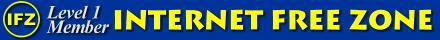Support free and non-commercial Internet.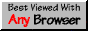This site works best in Any Browser, a campaign for non-specific WWW.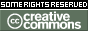This work is licensed under a Creative Commons License.